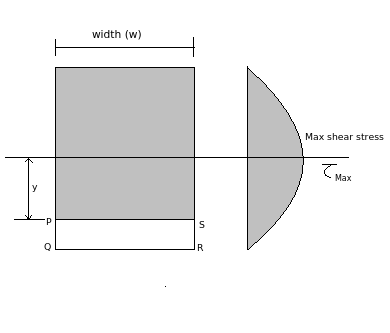# Shear stress on neutral axis of beam is maximum

Q.  The shear stress acting on the neutral axis of a beam is _____
- Published on 21 Sep 15

a. maximum
b. minimum
c. zero
d. none of the above

#### Discussion

• Sravanthi   -Posted on 24 Nov 15
- Maximum shear stress acts on the neutral axis of beam and is zero at both top and bottom surface.- Shear stress acting on the section PS is given as

τ = (SA y) / wI

Here, w is the width of section PS, A is the area of beam below y, y is the distance of centroid of shaded region from neutral axis and S is the shear force.

- When y is zero, shear stress is maximum, shear stress increases as y decreases and the variation of shear stress is parabolic as the equation is of 2nd degree.

## ➨ Post your comment / Share knowledgeEnter the code shown above:

(Note: If you cannot read the numbers in the above image, reload the page to generate a new one.)